# Basic course of matplotlib

Keywords: Javascript IPython REST

The original idea of doing this tutorial is: Although plt.plot(x,y) is a simple and convenient way, there are many detailed requirements for drawing tutorials involving academic paper, which need to further fine tune the pictures, and at this time, it needs to constantly Baidu Baidu, not to mention writing a tutorial to sort it out as a whole.
References:

https://zhuanlan.zhihu.com/p/93423829

https://matplotlib.org/1.5.1/faq/usage_faq.html#parts-of-a-figure

# Basic concepts

## 1. fig，ax，axes

Reference: https://matplotlib.org/1.5.1/faq/usage faq.htmlාparts-of-a-figure

• fig: Canvas object
• Axes (handle): Usage: ax = fig.add_subplot(1,1,1), for the case of subgraphs, each subplot is an axes
How to build axes according to fig is described in detail in ipynb of subplot
• Axis field, ax = fig.add [axes ([left, bottom, width, height])

Parameter Description: left, bottom, width, height percentage, i.e. proportion.

Axes and subplot are concepts at the same level. They both select a part of controllable area on the fig (canvas) to draw. The difference is that axes has a large degree of freedom, and you can specify the relevant parameters by yourself; while subplot divides fig at the same interval of format call. It can be said that subplot is a special case of axes.
For details, please refer to: https://www.zhihu.com/question/51745620
Refer to the figure below for details

## 2. Elements of image

[failed to transfer and save the pictures in the external link. The source station may have anti-theft chain mechanism. It is recommended to save the pictures and upload them directly (img-8g7h8HlT-1580022584091)(0matplotlib.jpg))

## Title Class

• Title, Title: ax.set_title()
• Title of X ﹐ label, y ﹐ label, XY coordinate axis: ax.set ﹐ xlabel ('x '), ax.set ﹐ ylabel ('y')

## Class axis

• Ax.set'aspect ('equal ') for horizontal and vertical axis equalization
• Range setting: ax.set Xlim (0,1), ax.set ylim (0,1)
• Grid setting: ax.grid (which ='minor ', axis ='both')

## Axis tick

This is probably one of the most common functions, because it often involves some axis adjustment

• Set the displayed position ax.set ﹣ [0,1,2]; ax.set ﹣ [0,1,2])
• Set the displayed position to the specified label ax.set ﹣ xticklabels (['a ','b','c '])
• Rotate label PLT. Setp (ax. Xaxis. Get jorticklabels(), rotation = - 45)
• Shift labels to the right
for tick in ax.xaxis.get_majorticklabels():
tick.set_horizontalalignment("right")

• Set other attributes of the label: font, facecolor, edgecolor
for label in ax.get_xticklabels() + ax.get_yticklabels():
label.set_fontsize(12)
#make the background a little clear, to clear the original data
label.set_bbox(dict(facecolor='blue', edgecolor='None', alpha=0.65 ))


• Primary and secondary tick marks, ax. Xaxis. Get ﹣ minor lines ()

Do not want to see the scale mark, the secondary scale line will not be displayed.

for line in ax.xaxis.get_minorticklines():
line.set_visible(False)


## Class spines

There are four controllable parameters: ax.spines['right'],ax.spines['left'],ax.spines['top'],ax.spines['bottom']
Select one of the following to explain, and the rest will expand by themselves

• Set visible ax. Spines ['right ']. Set visible (false)
• Set color ax. Spines ['right ']. Set [color ('b')
• Set "ticks" position ('bottom '), parameter left,right,top

This is common when the image size cannot be expanded, but there are many elements in the image, so if you can't put them down, you can put the ticks on top

• Set the origin of the coordinate axis as the center point ax. Spines ['left ']. Set Dou position (('data', 0))

ax.grid(True)

## annotate, annotation

ax.annotate(text,xy=(x,y))



text is the content, latex R '$XX$' is used, and XY parameter is the display position

# 3. Precautions:

• If you enter the latex formula in matplotlib, use the method of r'$xxx$'
• Set style, Matplotlib. Style. Use (u'grayscale '), xkcd, ggplot, classic
%matplotlib notebook
import matplotlib
from matplotlib import pyplot as plt

#%matplotlib tk
from matplotlib import rcdefaults
rcdefaults()
import numpy as np
import pandas as pd


# fig, subplot, axes

#Axes
# The axes command allows more manual placement of the plots in the figure.
fig = plt.figure(figsize= (6,4))
plt.show()

fig = plt.figure(figsize= (6,4))
plt.show()

fig = plt.figure(figsize= (6,4))
plt.show()

C:\ProgramData\Anaconda3\lib\site-packages\matplotlib\pyplot.py:522: RuntimeWarning: More than 20 figures have been opened. Figures created through the pyplot interface (matplotlib.pyplot.figure) are retained until explicitly closed and may consume too much memory. (To control this warning, see the rcParam figure.max_open_warning).
max_open_warning, RuntimeWarning)

<IPython.core.display.Javascript object>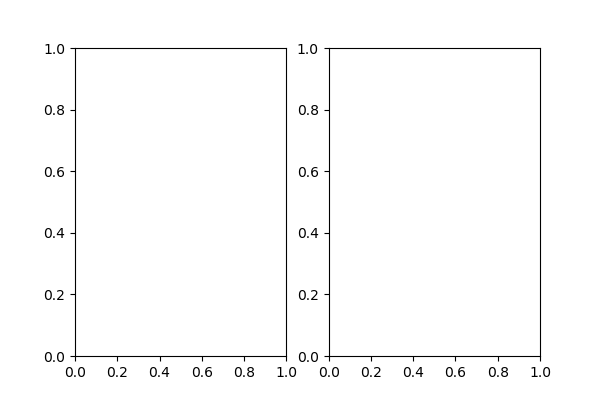<IPython.core.display.Javascript object>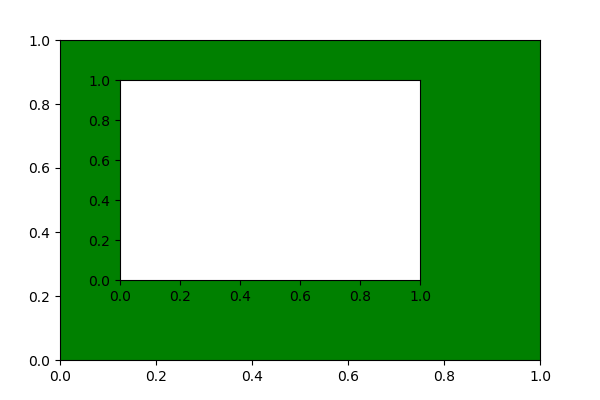<IPython.core.display.Javascript object>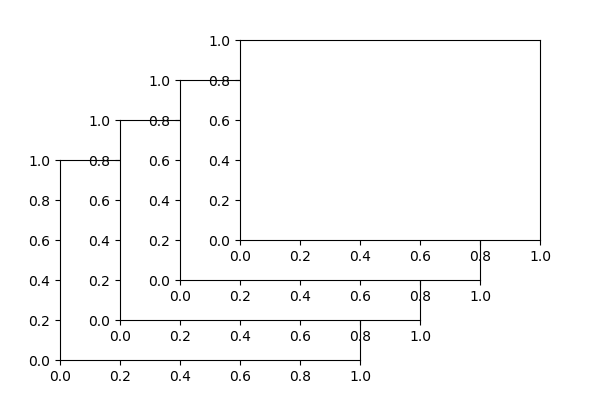# Figure basic setting diagram

# Numbers 0 - 256
X = np.linspace(-np.pi, np.pi, 256, endpoint=True)
# cosine(X), sin(X)
C, S = np.cos(X)+2, np.sin(X)

fig = plt.figure(figsize = (6,4))
axs = [ax1, ax2]

ax1.plot(X,C)
ax2.plot(C,S)

for ax in axs:
print(ax)
ax.set_title('test')
ax.set_xlabel('x'); ax.set_ylabel('y')

ax.set_xlim(np.min(X), np.max(X))
#ax.set_ylim

ax.set_yticks([0,1])
ax.set_xticks([-np.pi/2, 0, np.pi/2])
ax.set_xticklabels([r'-$\pi$',0, r'$\pi$' ])

ax.grid(True)

ax.annotate(r'$a$', xy = (0, 1),color="r",size=12)

ax1.annotate(r'$\cos(0) + 2 = 3$',
xy=(0, 3), xycoords='data',
xytext=(-90, -50), textcoords='offset points', fontsize=16,

plt.show()

AxesSubplot(0.125,0.11;0.352273x0.77)
AxesSubplot(0.547727,0.11;0.352273x0.77)

C:\ProgramData\Anaconda3\lib\site-packages\matplotlib\pyplot.py:522: RuntimeWarning: More than 20 figures have been opened. Figures created through the pyplot interface (matplotlib.pyplot.figure) are retained until explicitly closed and may consume too much memory. (To control this warning, see the rcParam figure.max_open_warning).
max_open_warning, RuntimeWarning)

<IPython.core.display.Javascript object>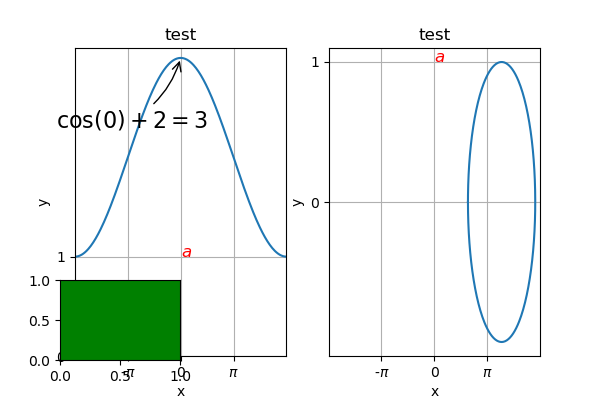# spines schematic

plt.figure(figsize = (6,4))
X = np.linspace(-np.pi, np.pi, 256, endpoint=True)
# cosine(X), sin(X)
C, S = np.cos(X), np.sin(X)
plt.plot(X, C, color="blue", linewidth=2.5)
plt.plot(X, S, color="red",  linewidth=2.5)

#Moving spines
#    Spines are the lines connecting the axis tick marks and noting the boundaries of the data area. They can be placed at arbitrary positions and until now, they were on the border of the axis. We'll change that since we want to have them in the middle. Since there are four of them (top/bottom/left/right), we'll discard the top and right by setting their visibility to False and we'll move the bottom and left ones to coordinate 0 in data space coordinates.
#    this is 2nd the axe preparation part
ax = plt.gca()   # to get the present axe
#ax.spines['right'].set_visible(False)
ax.spines['right'].set_color('b')
#ax.spines['top'].set_visible(False)
ax.spines['top'].set_color('y')

ax.xaxis.set_ticks_position('bottom')
ax.spines['bottom'].set_position(('data',0))
ax.spines['bottom'].set_visible(True)
#ax.spines['bottom'].set_color('g')

ax.yaxis.set_ticks_position('left')
ax.spines['left'].set_position(('data',0))
ax.spines['left'].set_visible(True)
ax.spines['left'].set_color('r')

plt.show()

<IPython.core.display.Javascript object>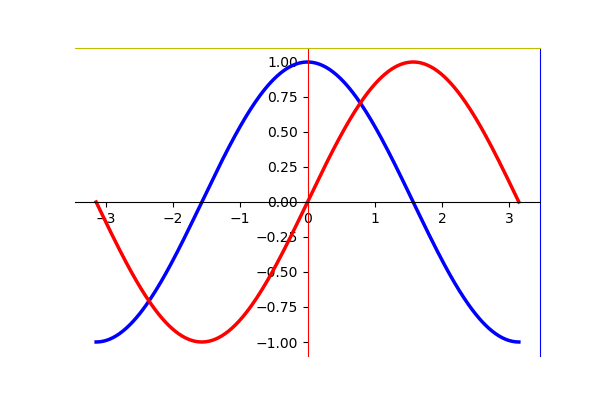Published 32 original articles, won praise and 10000 visitors+

Posted by fred2k7 on Sun, 26 Jan 2020 00:03:57 -0800